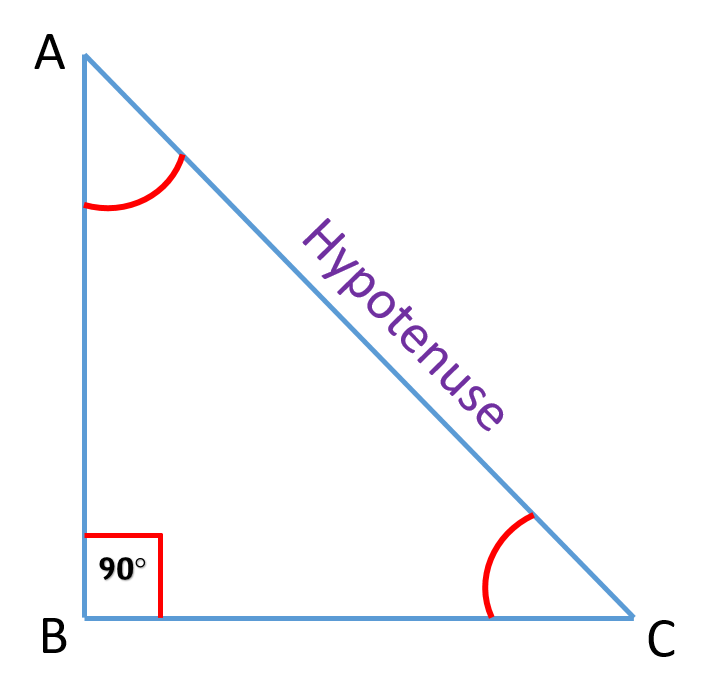# Pythagoras Theorem

Pythagoras Theorem states that -

### "In a right angle triangle, the square of the hypotenuse is equal to the sum of the squares of the other two sides".

Let us understand same using figure as follows:Pythagoras Theorem

Here, in $\ \triangle ABC$, $\ m \angle B = 90 \degree$,
$\therefore$ the side opposite to $\angle B$ will the hypotenuse (longest side).

The longest side of a right angle triangle is known as hypotenuse.

So, as per the theorem stated above,

\begin {aligned}\text{(Hypotenuse)}^2 &= \text{(side 1)}^2 + \text{(side 2)}^2 \\ \therefore AC^2 &= AB^2 + BC^2 \end {aligned}

Play with the Pythagorean triplets here.